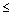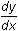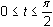Module 23 - Parametric Equations Introduction | Lesson 1 | Lesson 2 | Lesson 3 | Self-Test Self Test For Questions 1 - 5 use the parametric equations x = -sin(3t)*cos(t) y = -sin(3t)*sin(t) Graph the parametric equations for 0tin a [-2, 2] x [-1, 1] window. Findat t =/4. Findat t =/4. Find the equation of the tangent line to the parametric curve at t =/4. Find the length of the parametric curve forClick here to check your answers. < Back ©Copyright 2007 All rights reserved. | Trademarks | Privacy Policy | Link Policy